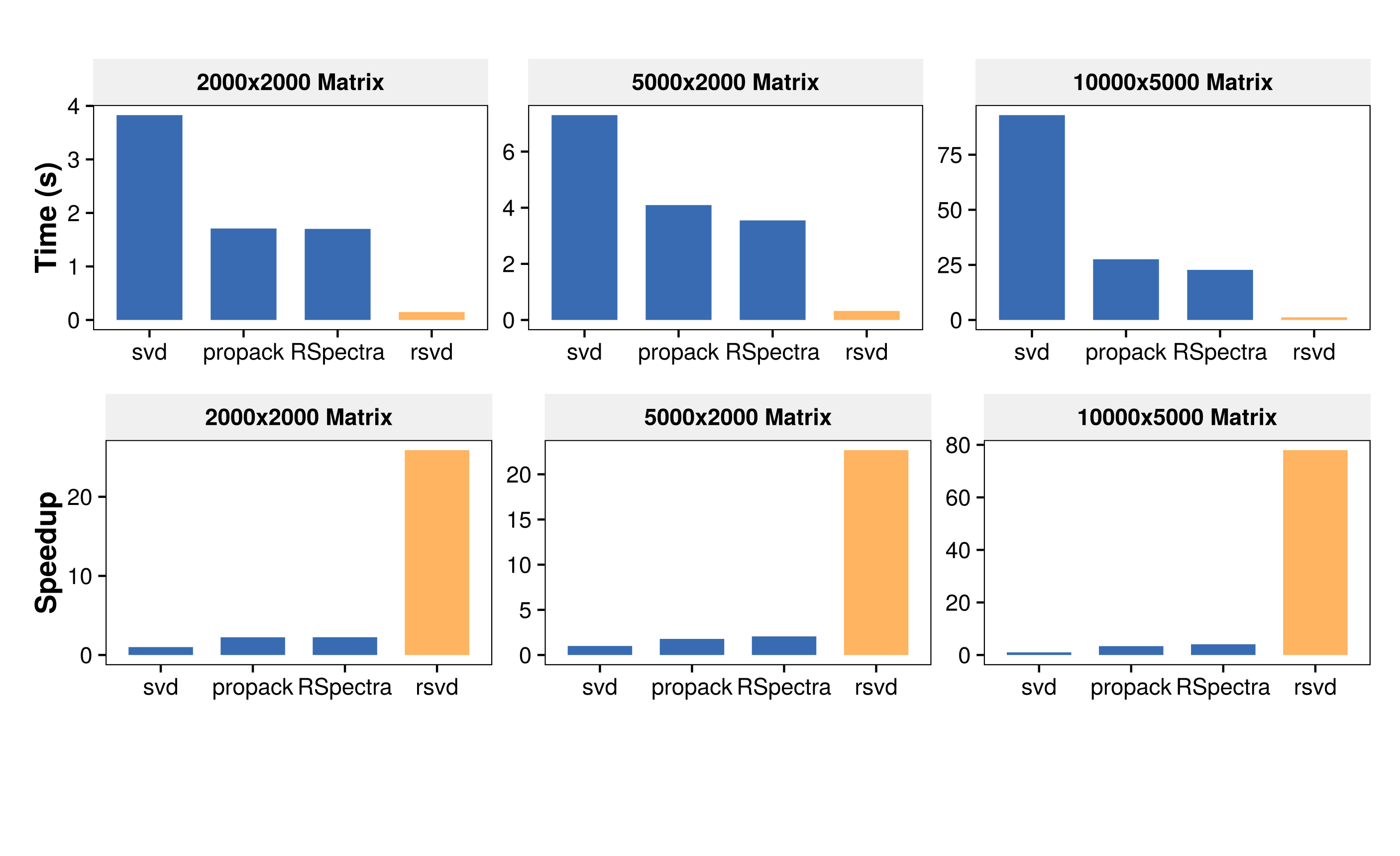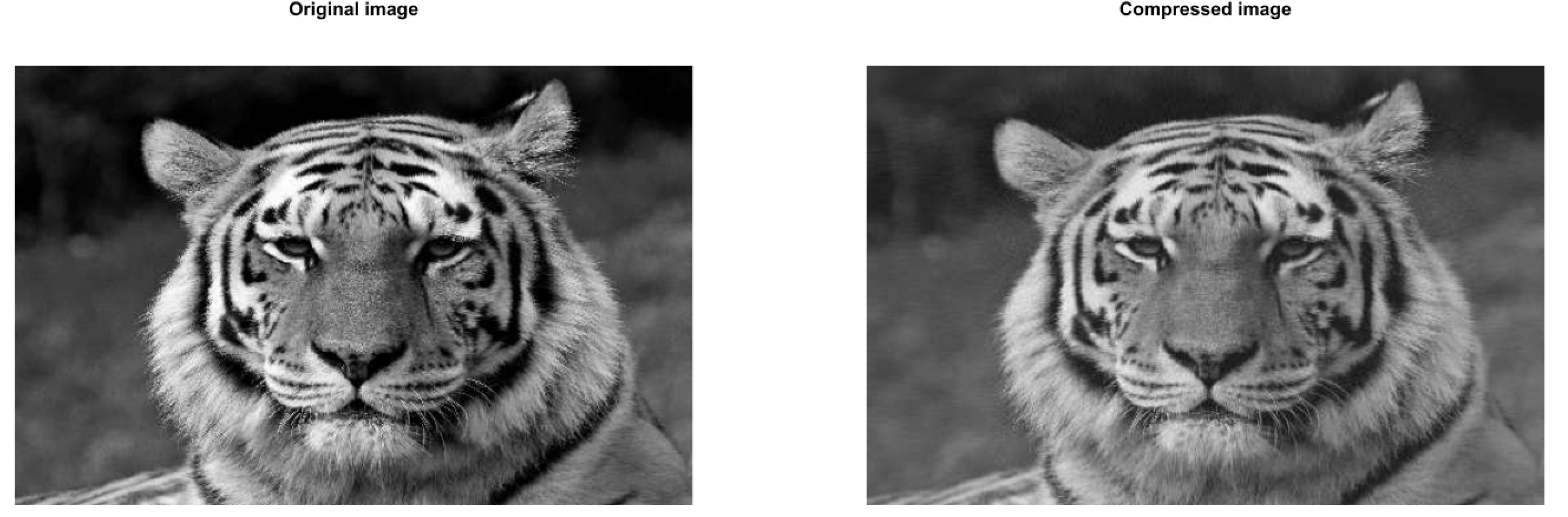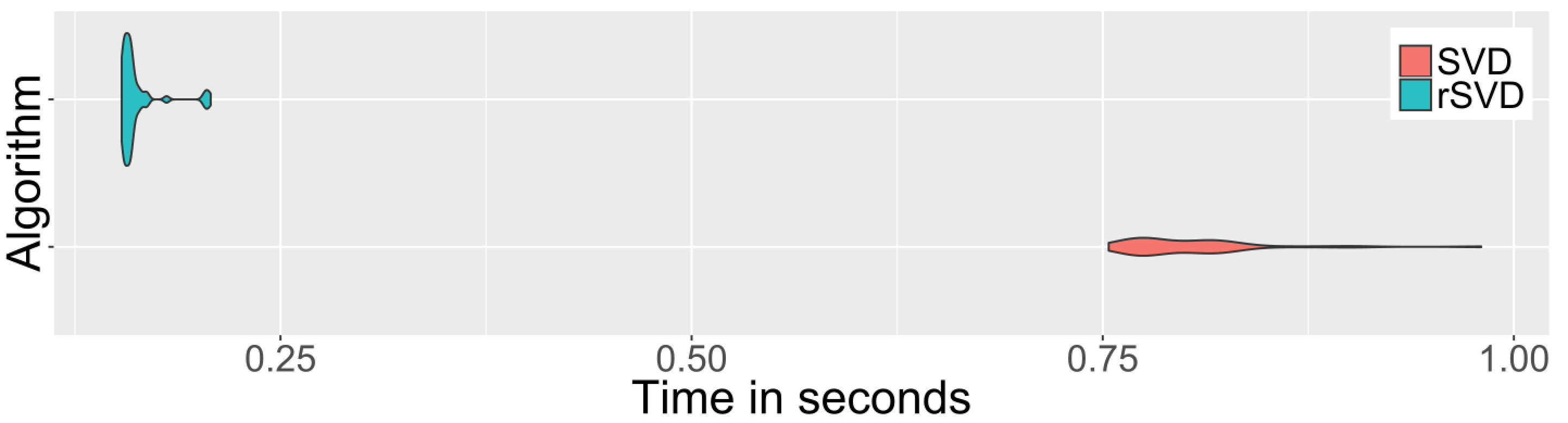# README.md In Benli11/rSVD: Randomized Singular Value Decomposition## Fast Randomized Singular Value Decomposition using R

Randomized singular value decomposition (rsvd) is a fast probabilistic algorithm that can be used to compute the near optimal low-rank singular value decomposition of massive data sets with high accuracy. The key idea is to compute a compressed representation of the data to capture the essential information. This compressed representation can then be used to obtain the low-rank singular value decomposition decomposition. The rsvd package provides one of the fastest routines for low-rank matrix approximations in R, as far as we know. The computational advantage becomes pronounced with an increasing matrix dimension (here target-rank k=50):The singular value decomposition plays a central role in data analysis and scientific computing. The SVD is also widely used for computing (randomized) principal component analysis (PCA), a linear dimensionality reduction technique. Randomized PCA (rpca) uses the approximated singular value decomposition to compute the most significant principal components. This package also includes a function to compute (randomized) robust principal component analysis (RPCA). In addition several plot functions are provided. See for further details: “Randomized Matrix Decompositions using R”.

## SVD example: Image compression

``````library(rsvd)
data(tiger)

# Image compression using randomized SVD
s <- rsvd(tiger, k=150)
tiger.re = s\$u %*% diag(s\$d) %*% t(s\$v) # reconstruct image

# Display orginal and reconstrucuted image
par(mfrow=c(1,2))
image(tiger, col = gray((0:255)/255))
image(tiger.re, col = gray((0:255)/255))
``````

Here are the results:and the speedup gained over the base SVD function:

``````library(microbenchmark)

timing_svd <- microbenchmark(
'SVD' = svd(tiger, nu=150, nv=150),
'rSVD' = rsvd(tiger, k=150),
times=50)

print(timing_svd, unit='s')
``````## Installation

Install the rsvd package via CRAN

``````install.packages("rsvd")
``````

You can also install the development version from GitHub using devtools:

``````devtools::install_github("erichson/rsvd")
``````

The source packge can be obtained here: CRAN: rsvd.

## New in Version 1.0.0

• Support for non-default matrix types to deal with large-scale matrices that are held on file, added by Aaron Lun.
• Fixed a bug which occured runninig rpca with k=1 and retx=TRUE, discovered by Will.

## References

Benli11/rSVD documentation built on Nov. 6, 2018, 10:46 p.m.